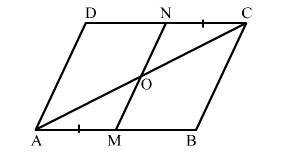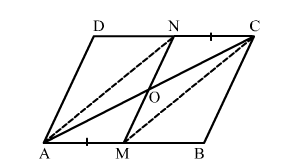# In a parallelogram ABCD, points M and N have been taken on opposite sides AB and CD respectively such that AM = CN.

Question:

In a parallelogram ABCD, points M and N have been taken on opposite sides AB and CD respectively such that AM CN. Show that AC and MN bisect each other.Solution:Given: In a parallelogram ABCD, AM CN.

To prove: AC and MN bisect each other.

Construction: Join AN and MC.

Proof:
Since, ABCD is a parallelogram.

$\Rightarrow A B \| D C$

$\Rightarrow A M \| N C$

Also, AM = CN           (Given)

Thus, AMCN is a parallelogram.

Since, diagonals of a parallelogram bisect each other.

Hence, AC and MN bisect each other.